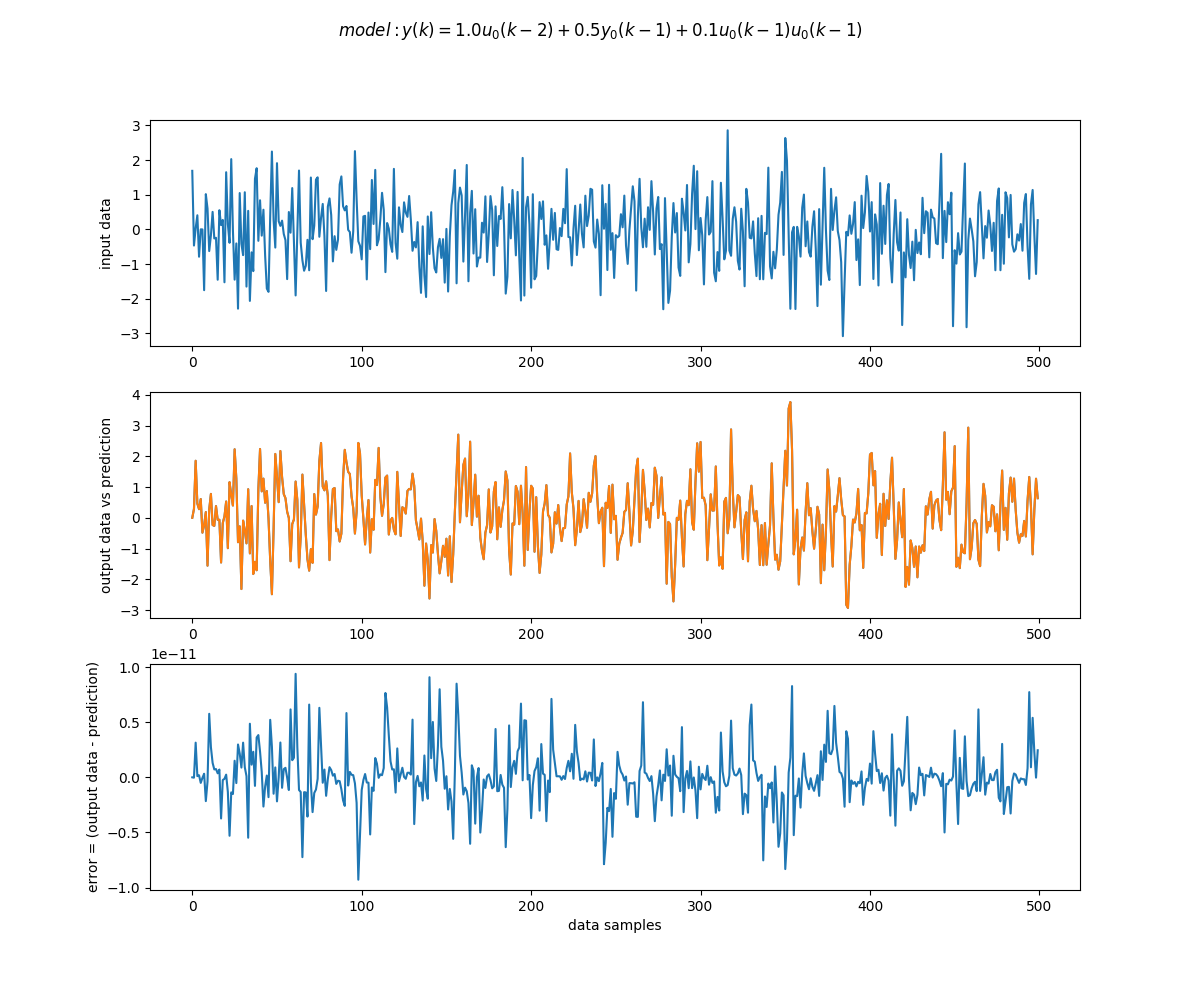## Introduction

The SISONARMAX algorithm finds a mathematical model in the form of a discrete difference equation for any given set of input-output data. This model can be used to make predictions.

SISONARMAX is the polynomial expansion of the Nonlinear AutoRegresive Moving Average model with eXogenous Inputs.This implementation can handle one input and one ouput and no noise model is generated.

## Algorithm Usage

The algorithm requires:

1. 'inputdata'. Valid path to a .csv file, containing the recorded input of the system (u).
2. 'outputdata'. Valid path to a .csv file, containing the recorded output of the system (y).
3. 'location': Valid path where the generated file(s) will be saved.

Optional parameters used in the model obtention process. Default values shown in parenthesis:

• 'max_reg(3)': Maximum number of polynomial terms included in the model.
• 'deg(1)': Polynomial degree of the model.
• 'in_max_lag(3)': Maximum number of delayed input versions that will be considered.
• 'out_max_lag(3)': Maximum number of delayed output version that will be used in the model.

The algorithm returns

If a model was obtained successfully:

1. The model description in the form of a difference equation display in the console.
2. 'prediction.csv' stored in 'location' containing the values of the predicted output using the obtained model
3. 'prediction.png', a figure stored in the 'location' which shows:
• a) Given input data
• b) Output data vs Prediction
• c) Error between Output data and Prediction

## Example

Input:

``````{"inputdata":"data://cluna7/test_data/input_example.csv",
"outputdata":"data://cluna7/test_data/output_example.csv",
"max_reg":3,
"deg": 2,
"in_max_lag": 3,
"out_max_lag": 3,
"location":"data://.algo/cluna7/SISONARMAX/temp/"
}``````

Ouput:

``````{
"model": "y(k)=1.0u_0(k-2)+0.5y_0(k-1)+0.1u_0(k-1)u_0(k-1)"
}``````Contents